Courses

# Olympiad Test : Puzzle Test - 2

## 20 Questions MCQ Test Science Olympiad for Class 5 | Olympiad Test : Puzzle Test - 2

Description
This mock test of Olympiad Test : Puzzle Test - 2 for Class 5 helps you for every Class 5 entrance exam. This contains 20 Multiple Choice Questions for Class 5 Olympiad Test : Puzzle Test - 2 (mcq) to study with solutions a complete question bank. The solved questions answers in this Olympiad Test : Puzzle Test - 2 quiz give you a good mix of easy questions and tough questions. Class 5 students definitely take this Olympiad Test : Puzzle Test - 2 exercise for a better result in the exam. You can find other Olympiad Test : Puzzle Test - 2 extra questions, long questions & short questions for Class 5 on EduRev as well by searching above.
QUESTION: 1

### Study the given information and answer the questions that follow: P, Q, R, S, T, U and V are sitting on a wall and all of them are facing east. R is on the immediate right of S. Q is at the extreme end and has T as his neighbour. S is sitting third from the south end. V is between T and U. Q. ​Who is sitting to the right of T?

Solution:

R is to the right of S. V is between T and U. S is third from South direction. So Q will be at the extreme end from North direction because Q has T as its neighbour.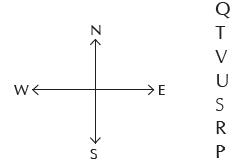V is sitting to the right of T.

QUESTION: 2

### Study the given information and answer the questions that follow: P, Q, R, S, T, U and V are sitting on a wall and all of them are facing east. R is on the immediate right of S. Q is at the extreme end and has T as his neighbour. S is sitting third from the south end. V is between T and U. Q. Immediately between which of the following pairs of people is S sitting?

Solution:

R is to the right of S. V is between T and U. S is third from South direction. So Q will be at the extreme end from North direction because Q has T as its neighbour.Clearly, S is sitting between U and R.

QUESTION: 3

### Study the given information and answer the questions that follow: P, Q, R, S, T, U and V are sitting on a wall and all of them are facing east. R is on the immediate right of S. Q is at the extreme end and has T as his neighbour. S is sitting third from the south end. V is between T and U. Q. Which of the following pairs of people are sitting at the extreme ends?

Solution:

R is to the right of S. V is between T and U. S is third from South direction. So Q will be at the extreme end from North direction because Q has T as its neighbour.It is clear from figure, Q and P are sitting at the extreme ends.

QUESTION: 4

​Study the given information and answer the questions that follow:
P, Q, R, S, T, U and V are sitting on a wall and all of them are facing east. R is on the immediate right of S. Q is at the extreme end and has T as his neighbour. S is sitting third from the south end. V is between T and U.
Q. Jai, Khyati, Kajal, Huma, Dhairya are sitting in a row. Jai is on the left of the person sitting in the centre but is on the right of Khyati. Huma is on the right of Kajal. Dhairya is on the right of Huma. Dhairya is the second person from the person sitting in the centre. Who is the person sitting in the centre?

Solution:

R is to the right of S. V is between T and U. S is third from South direction. So Q will be at the extreme end from North direction because Q has T as its neighbour.The order of sitting is – Khyati, Jai, Kajal, Huma, Dhairya So, Kajal is sitting at the centre.

QUESTION: 5

​Study the given information and answer the questions that follow:
P, Q, R, S, T, U and V are sitting on a wall and all of them are facing east. R is on the immediate right of S. Q is at the extreme end and has T as his neighbour. S is sitting third from the south end. V is between T and U.
Q. Six books P, Q, R, S, T and U are placed on a table. Q, R, T and U have green covers while others have yellow covers. P, S and Q are new books while the rest are old books. P, R and Q are related to law and rest other books are related to Medical. Which two books are old medical books and have green covers?

Solution: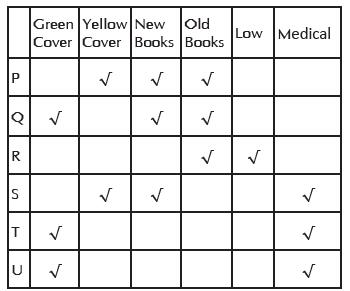It is clear from the table that T and U are old books related to Medical and have green covers.

QUESTION: 6

Study the following information and answer the questions given below: In a class there are six boys H, I, J, K, L, M and five girls P, Q, R and T. For a sports team, six people are to be selected under the following conditions:
(1) H and K should be together.
(2) J should not go with S.
(3) S and T should be together.
(4) J and Q should be together.
(5) I and R should be together.
(6) K should not go with P.
(7) I should not go with L.
Q. If there are five boys in the team,the lone girl team member is

Solution:

Boys H, I, J, K, L and M, I and L cannot go together. So two groups H I J K M and H J K M L are possible. As I and R should be together and J and Q should be together. Hence, H L J K M Q is the correct answer and Q is the lone girl member.

QUESTION: 7

​Study the following information and answer the questions given below: In a class there are six boys H, I, J, K, L, M and five girls P, Q, R and T. For a sports team, six people are to be selected under the following conditions:
(1) H and K should be together.
(2) J should not go with S.
(3) S and T should be together.
(4) J and Q should be together.
(5) I and R should be together.
(6) K should not go with P.
(7) I should not go with L.
Q. If four members have to be girls, the members of the team are:

Solution:

I, M, P,R, S and T will be the team members.

QUESTION: 8

​Study the following information and answer the questions given below: In a class there are six boys H, I, J, K, L, M and five girls P, Q, R and T. For a sports team, six people are to be selected under the following conditions:
(1) H and K should be together.
(2) J should not go with S.
(3) S and T should be together.
(4) J and Q should be together.
(5) I and R should be together.
(6) K should not go with P.
(7) I should not go with L.
Q. If four members including L have to be boys, the members other than L are

Solution:

H, K, L, F, S and T will be the team members.

QUESTION: 9

​Study the following information and answer the questions given below: In a class there are six boys H, I, J, K, L, M and five girls P, Q, R and T. For a sports team, six people are to be selected under the following conditions:
(1) H and K should be together.
(2) J should not go with S.
(3) S and T should be together.
(4) J and Q should be together.
(5) I and R should be together.
(6) K should not go with P.
(7) I should not go with L.
Q. If, including P, the team has three girls, the members other than P are

Solution:

P I J M Q and R will be the team members.

QUESTION: 10

​​Study the following information and answer the questions given below: In a class there are six boys H, I, J, K, L, M and five girls P, Q, R and T. For a sports team, six people are to be selected under the following conditions:
(1) H and K should be together.
(2) J should not go with S.
(3) S and T should be together.
(4) J and Q should be together.
(5) I and R should be together.
(6) K should not go with P.
(7) I should not go with L.
Q. If Rohan works on all Saturdays and the dates which are multiples of 5 are considered as holidays, then how many days is he working? (Assume that Sunday is a holiday) (IMO 2013)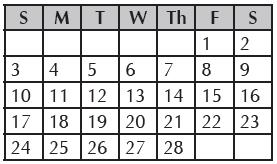Solution:

Total holidays = 4 Sundays + multiple of 5 dates. 4 Sundays + 5, 15, 20, 25 4 + 4 = 8 days Working days = 28 - 8 = 20 days.

QUESTION: 11

A scrap dealer took of weight five waste items,
P1, P2, P3, P4, and P5
(1) P1 weighs twice as much as P2
(2) P2 weighs four and a half times as
much as P3
(3) P3 weighs half as much as P4
(4) P5 weighs less than P, but more
than P3
(5) P4 weighs half as much as P5.
Q. Which of the following represents the descending (decreasing) order of weights of the items?

Solution:

(C) P1 = 2P2, P2 =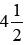, P3, P3 =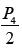P1 > P5 > P3
P4 =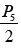P1> P2 > P5 > P3> P4

QUESTION: 12

A scrap dealer took of weight five waste items,
P1, P2, P3, P4, and P5
(1) P1 weighs twice as much as P2
(2) P2 weighs four and a half times as
much as P3
(3) P3 weighs half as much as P4
(4) P5 weighs less than P, but more
than P3
(5) P4 weighs half as much as P5.
Q. Which of the following is lightest in weight?

Solution:

P4 is the lightest.

QUESTION: 13

A scrap dealer took of weight five waste items,
P1, P2, P3, P4, and P5
(1) P1 weighs twice as much as P2
(2) P2 weighs four and a half times as
much as P3
(3) P3 weighs half as much as P4
(4) P5 weighs less than P, but more
than P3
(5) P4 weighs half as much as P5.
Q. Which of the following is heaviest in weight?

Solution:

P1 is the heaviest.

QUESTION: 14

A scrap dealer took of weight five waste items,
P1, P2, P3, P4, and P5
(1) P1 weighs twice as much as P2
(2) P2 weighs four and a half times as
much as P3
(3) P3 weighs half as much as P4
(4) P5 weighs less than P, but more
than P3
(5) P4 weighs half as much as P5.
Q. ​Which two items are heavier than P5?

Solution:

P1 and P2 are heavier than P5.

QUESTION: 15

A scrap dealer took of weight five waste items,
P1, P2, P3, P4, and P5
(1) P1 weighs twice as much as P2
(2) P2 weighs four and a half times as
much as P3
(3) P3 weighs half as much as P4
(4) P5 weighs less than P, but more
than P3
(5) P4 weighs half as much as P5.
Q. Which two articles are lighter than P5

Solution:

P3 and P4 are lighter than P5.

QUESTION: 16

A scrap dealer took of weight five waste items,
P1, P2, P3, P4, and P5
(1) P1 weighs twice as much as P2
(2) P2 weighs four and a half times as
much as P3
(3) P3 weighs half as much as P4
(4) P5 weighs less than P, but more
than P3
(5) P4 weighs half as much as P5.
Q. Ram, Shyam, Shiv, Krishna, Sudhir and Subhash are students of a course. Ram Knows more than Krishna. Shyam knows as much as Sudhir. Shiv knows less than Subhash. Krishna knows more than Shyam. The best knowledgeable person among all students is

Solution:

Ram > Krishna, Shyam = Sudhir Subhash > Shiv; Krishna > Shyam Order in possessing Knowledge – Ram > Krishna > Shyam = Sudhir > Subhash > Shiv So, Ram is the most Knowledgeable person.

QUESTION: 17

A * B = A and B are of same age.
A - B = B is younger than A.
A + B = A is younger than B.
Q. Amit * Monika - Raju means

Solution:

Amit ∗ Monika means Amit and Monika have same age. Monika - Raju means Raju is younger than Monika and Amit. So, Raju is the youngest.

QUESTION: 18

A * B = A and B are of same age.
A - B = B is younger than A.
A + B = A is younger than B.
Q. ​X + Y + Z is same as

Solution:

X + Y = X < Y; Y + Z = Y < Z
So X < Y < Z
Z - Y = Y< Z;
Y - X = X < Y
So X < Y < Z
∴ X + Y + Z = Z - Y - X

QUESTION: 19

A * B = A and B are of same age.
A - B = B is younger than A.
A + B = A is younger than B.
Q. Five girls are sitting in a row. Reshma is adjacent to Mona. Mona is at the middle in the row. Divya is not adjacent to Sushma. Reshma is not adjacent to Sushma or Manorama. Divya is adjacent to whom out of the following.

Solution:

The order of sitting all the five girls is Divya, Reshma, Mona, Sushma, Monorama. So, Reshma is adjacent to Divya.

QUESTION: 20